The principal goal of this precept is to review the conceptual framework behind computing p-value and hypothesis testing using analytic methods. The steps are:

• Compute a statistic (e.g., the sample mean, or a t-statistic)
• Figure out how that statistic would be distributed if the null hypothesis were true
• Figure out fraction of the time that the statistic would be as extreme or more extreme than what we observe, if the null hypothesis were true
• This is the p-value. We can compare it to a threshold. If the p-value is smaller than a threshold, we can conclude that

We will load the finches example, and compute some summary statistics:

library(Sleuth3)
finches <- case0201
finches %>% group_by(Year) %>%
summarize(count = n(), mean = mean(Depth), sd = sd(Depth))
## # A tibble: 2 x 4
##    Year count  mean    sd
##   <int> <int> <dbl> <dbl>
## 1  1976    89  9.47 1.04
## 2  1978    89 10.1  0.906

### Problem 1: the z-statistic

#### Problem 1(a)

Let’s concentrate on finches in 1976. Repeatedly generate datasets of 89 measurements with mean 9.47 and standard deviation 1.04. Display the histogram of the measurements. For example, if you generate 10,000 datasets, you should have a vector with 10,000 sample means.

The point of the problem is to understand the setting in which p-values are computed: we imagine repeating the experiment many times

##### Solution
get.sample.mean <- function(){
mean(rnorm(n = 89, mean = 9.47, sd = 1.04))
}

means <- replicate(10000, get.sample.mean())
df <- data.frame(mean = means)
ggplot(df) +
geom_histogram(mapping = aes(x = mean), bins = 30)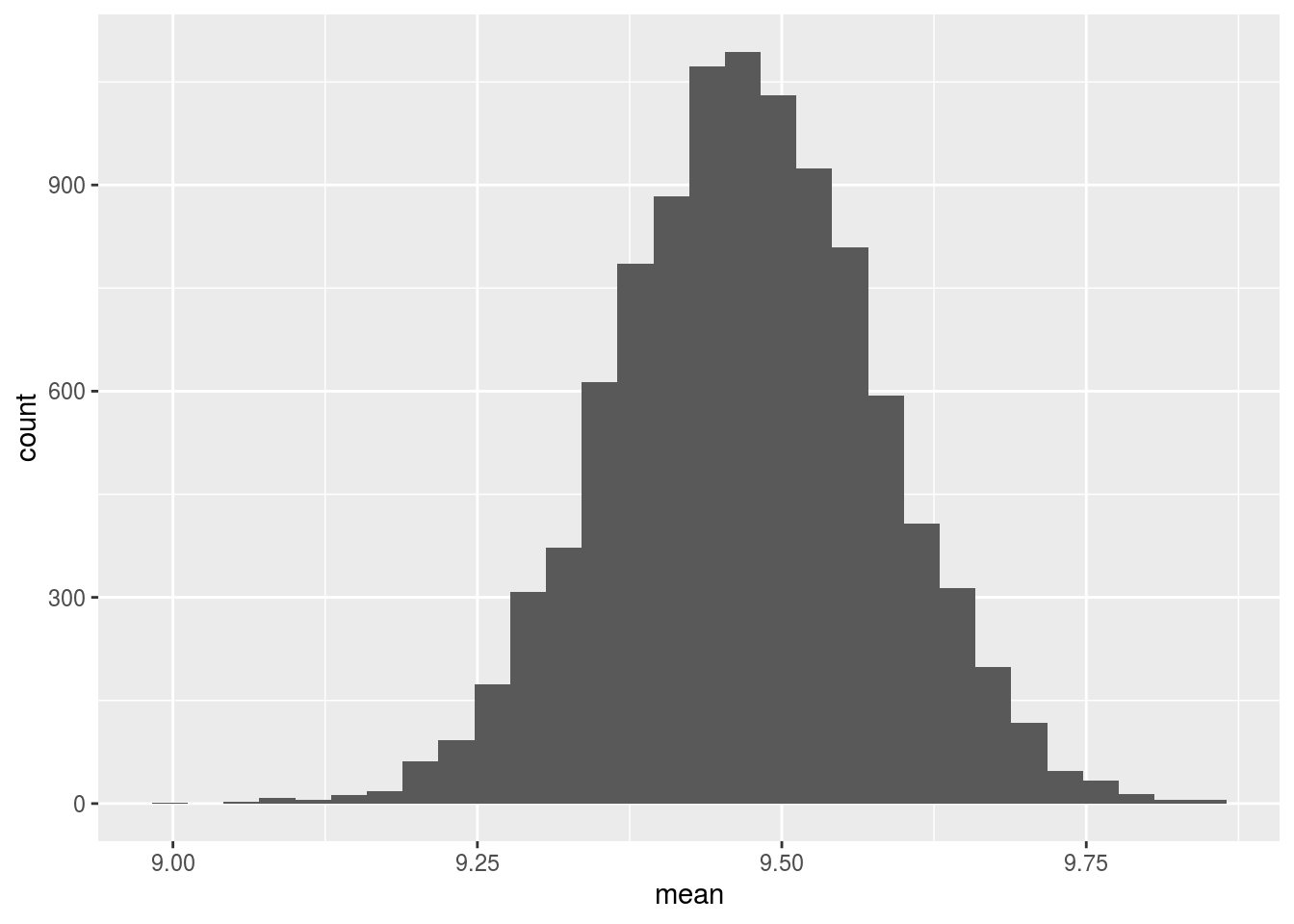#### Problem 1(b)

State what the distrbution of the sample mean $$\bar{X}$$ is.

The point of the problem is to understand sampling distributions. Here, we are thinking about how $$\bar{X}$$ can change with repeated experiments, and expressing it as a formula.

##### Solution

$\bar{X} \sim N(9.47, 1.04^2/89) = N(9.47, 0.11^2)$

#### Problem 1(c)

Use pnorm in order to compute the fraction of the measurements in an individual sample (i.e., in an individual experiment with 89 measurements) that you’d expect to be below 9.3

The point of the problem is to connect the use of pnorm to the distribution of the random variable.

pnorm(q = 9.3, mean = 9.47, sd = 1.04)
##  0.4350775

#### Problem 1(d)

Use pnorm in order to compute the fraction of the sample means that you’d expect to be below 9.3.

The point of the problem is to see the distinction between the distribution of an individual measurement and the distribution of the sample mean. The answers in 1(c) and 1(d) are different.

pnorm(q = 9.3, mean = 9.47, sd = 0.11)
##  0.06111818

#### Problem 1(e)

Using the simulated samples, compute the fraction of the sample means that are below 9.3. You should expect roughly the same answer as in Problem 1(d).

The point of the problem is to understand that we can compute pnorm using a simulation

mean(means < 9.3)
##  0.0602

#### Problem 1(f)

You can compute the z-statistic using $z\sim \frac{\bar{X}-\mu}{\sigma/\sqrt{n}}$

Compute the z-statistics for each sample mean.

Display the histogram of the z-statistics.

The point of the problem is to connect the z-statistics you get empirically to the distribution $$N(0, 1)$$, which is the distribution of the z-statistics assuming $$\mu$$ is the true mean of the population.

##### Solution
z <- (means-9.47)/0.11
df <- data.frame(z = z)
ggplot(df) +
geom_histogram(mapping = aes(x = z), bins = 30)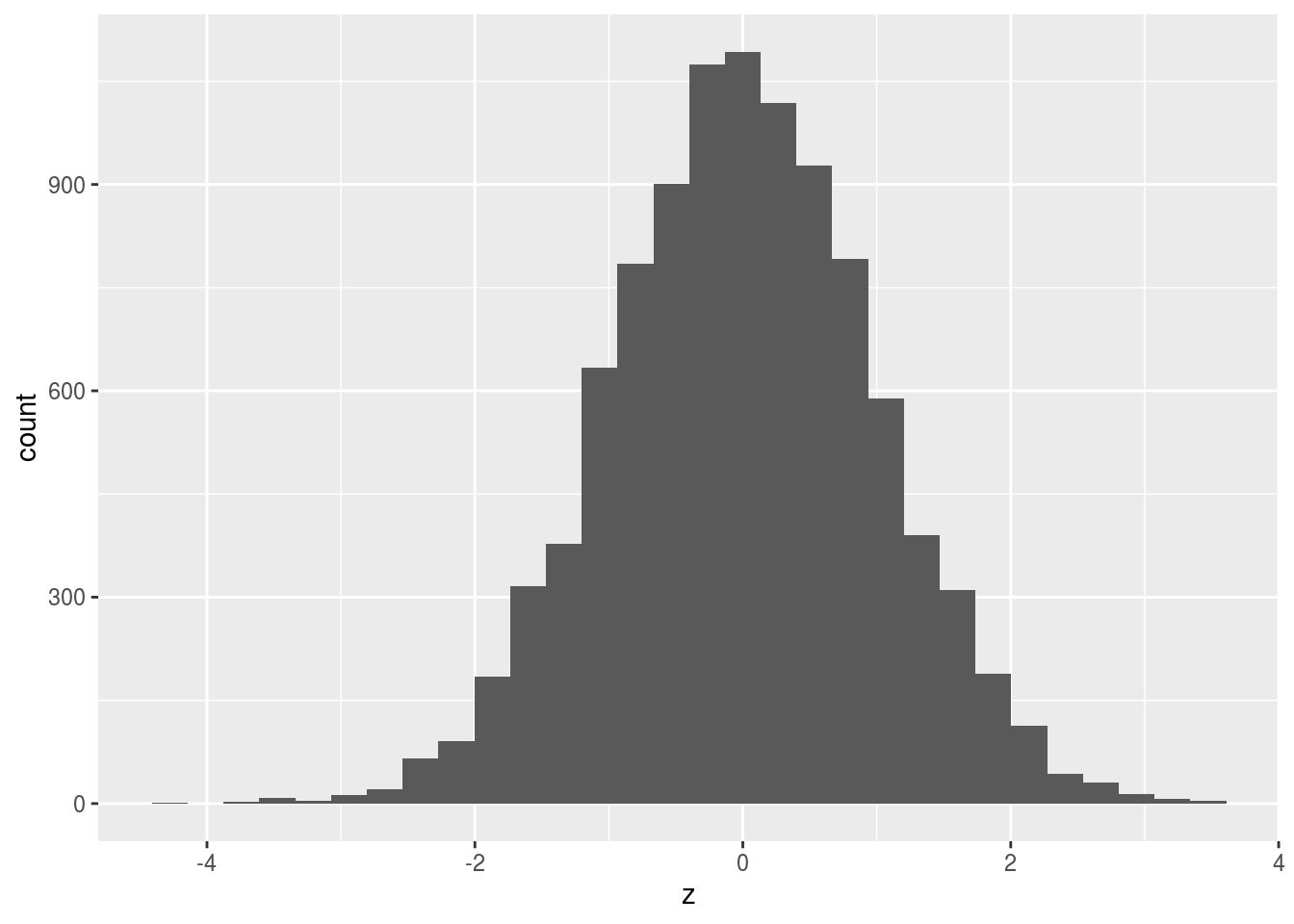#### Problem 1(g)

Use qnorm(..., mean = 0, sd = 1) to get the value of the z statistic q such that $$P(z < q)$$ corresponds to the answers to 1(c) and 1(d).

The point of the problem is to connect pnorm and qnorm

##### Solution

qnorm(p = 0.4350775, mean = 0, sd = 1)

#### Problem 1(g)

Get the answer to 1(f) without using qnorm

The point of the problem is to practice using the fact that if $$X\sim N(0, 1)$$, then $$(aX+b)\sim N(b, a^2)$$, and if $$Y\sim N(\mu, \sigma^2)$$ then $$\frac{Y-\mu}{\sigma}\sim N(0, 1)$$

##### Solution

$z = \frac{9.3-9.47}{1.04}$

$z = \frac{9.3-9.47}{0.11}$

### Problem 2: the t-statistic

Use the same simulation as in Problem 1, with mean $$\mu = 9.47$$ and standard deviation $$\sigma = 1.04$$. For each sample, compute the t-statistic

$\frac{\bar{X}-\mu}{s/\sqrt{n}}, s = sd(X)$

Display the histogram of the t-statistics.

Compute the probability that the t-statistic is smaller than -1, both using pt and using the simulated data.

The point of the problem is to understand the distribution of the t-statistic. You should see that it’s disributed according to a t-Distribution, which looks similar to the Normal distribution

##### Solution
get.t <- function(){
sample <- rnorm(n = 89, mean = 9.47, sd = 1.04)
(mean(sample) - 9.47)/(sd(sample)/sqrt(89))
}

t.stats <- replicate(10000, get.t())
df <- data.frame(t.stat = t.stats)
ggplot(df) +
geom_histogram(mapping = aes(x = t.stat), bins = 30)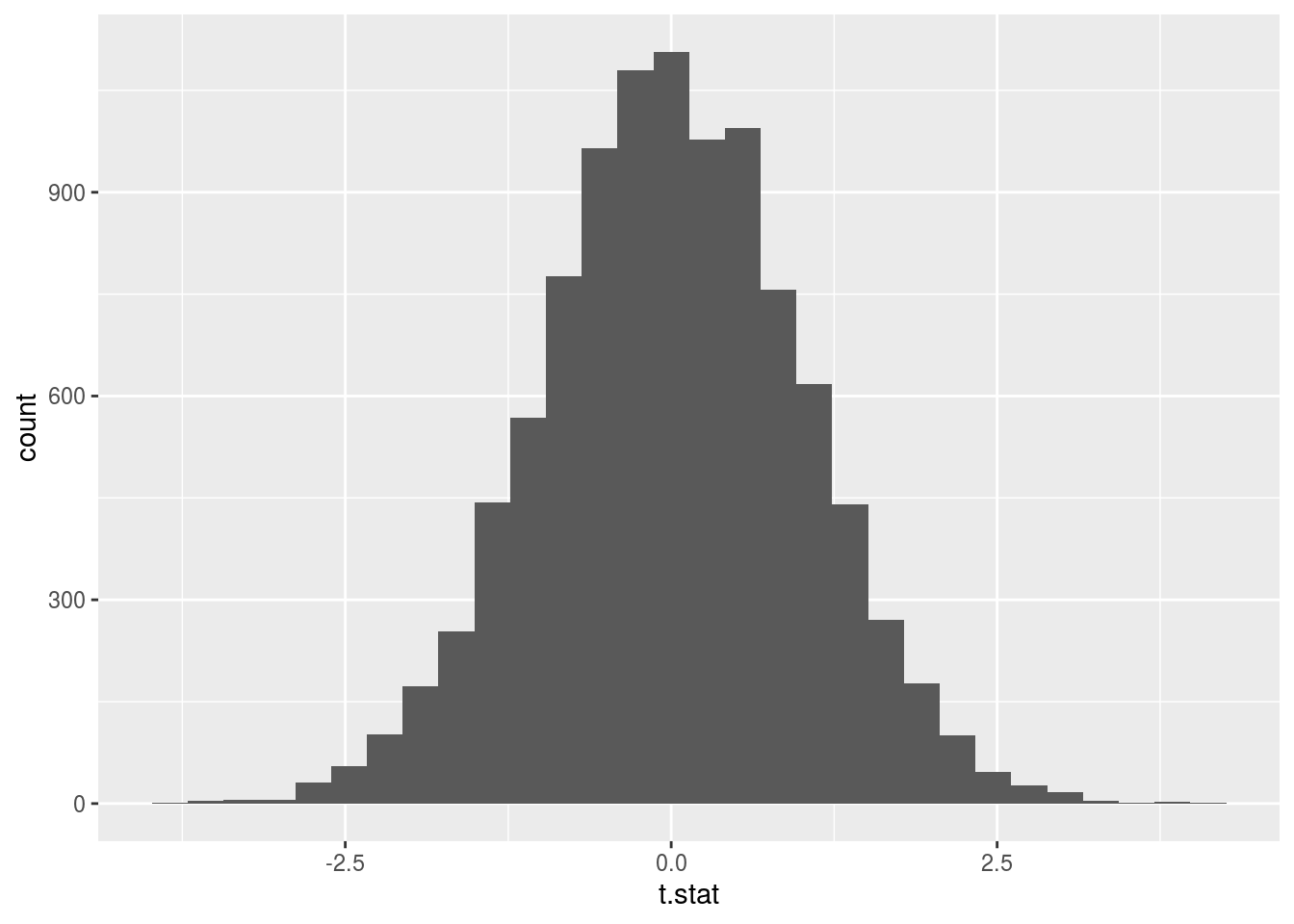mean(t.stats < -1)
##  0.155
pt(q = -1, df = 88)
##  0.1600262

### Problem 3: the t-statistic when the null hypothesis is false

Use the same simulation, with the mean 9.47, but now compute the t-statistic with $$\mu = 8.5$$.

Display the histogram of the t-statistics you computed. Note that this is not a histogram of a t-distribution.

What is the most frequent t-statistic $$t$$ that you observe? What is the probability of observing a t-statistic that is as far as $$t$$ is or farther from 0? For what null-hypothesis did you just compute a p-value?

The point of this problem is to understand a subtle point: the t-statistic is only t-distributed if the null hypothesis is true. If it is not, the t-statistic is not t-distributed. If the hypothesis is false, the t-statistic will not anymore have a mean 0

##### Solution
get.t.diff.mu <- function(){
sample <- rnorm(n = 89, mean = 9.47, sd = 1.04)
(mean(sample) - 8.5)/(sd(sample)/sqrt(89))
}

t.stats <- replicate(n = 100000, get.t.diff.mu())
df <- data.frame(t.stat = t.stats)
ggplot(df) +
geom_histogram(mapping = aes(x = t.stat), bins = 30)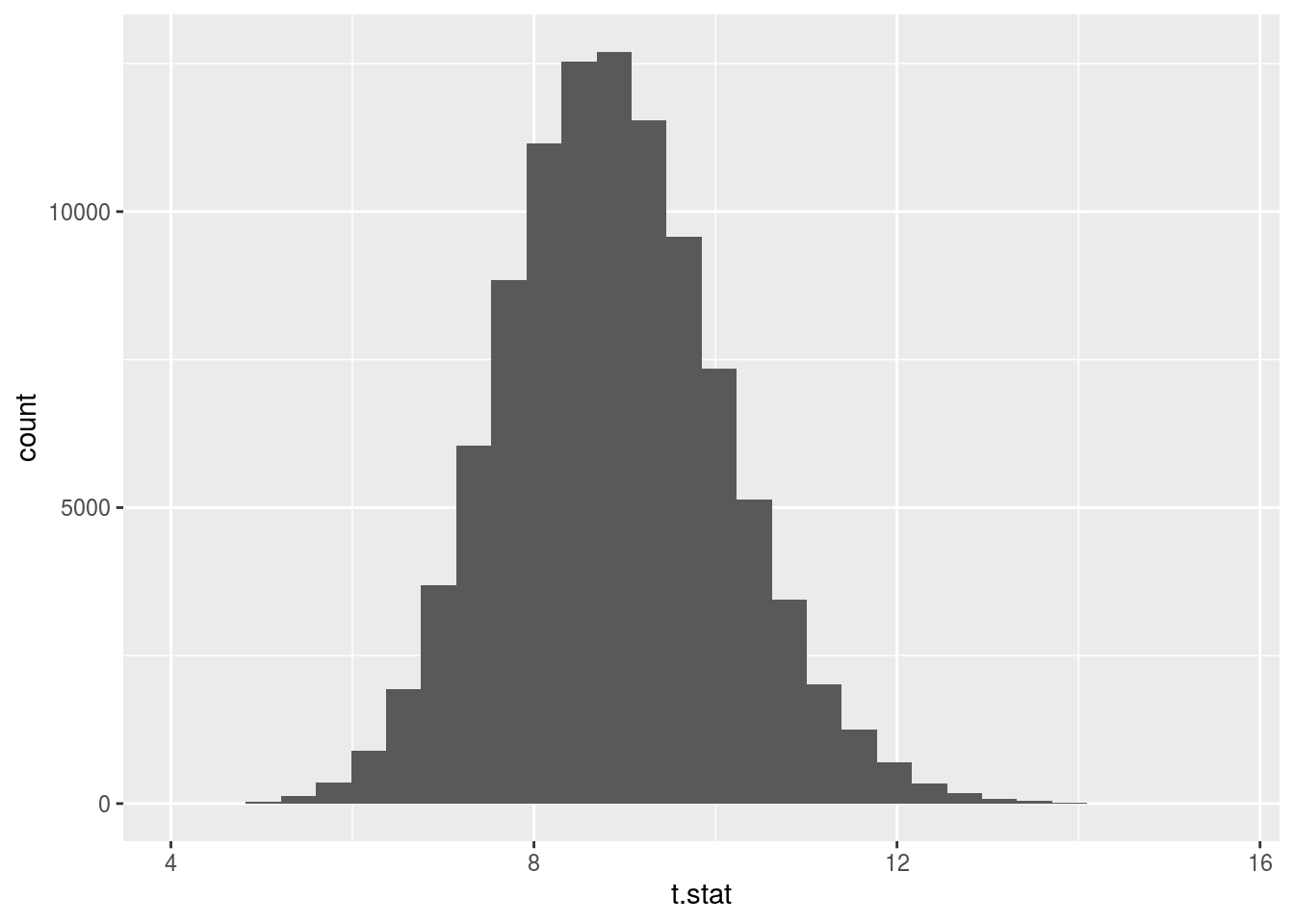The mode is around 8.8.

t <- (8.8-9.47)/(1.04/sqrt(89))
2 * pt(-abs(t), df = 88)
##  3.054393e-08

The null hypothesis is that $$\mu = 9.47$$

### Problem 4: The two-sample test

Generate data for both 1976 and 1978, repeatedly, with a mean of 9.8 and sd of 1.0 for both 1976 and 1978. For each sample, compute

$t = \frac{\bar{X_2}-\bar{X_1}}{\sqrt{\frac{s_1^2}{n_1} + \frac{s_2^2}{n_2}}}$

Display the histogram of the t statistics.

Compute the t-statistic using the data from

finches %>% group_by(Year) %>%
summarize(count = n(), mean = mean(Depth), sd = sd(Depth))
## # A tibble: 2 x 4
##    Year count  mean    sd
##   <int> <int> <dbl> <dbl>
## 1  1976    89  9.47 1.04
## 2  1978    89 10.1  0.906

Use both pt and the vector of t-statistics you generate to test the null hypothesis that the means in 1976 and 1978 are the same.

The point of the problem is to practice the connection between the anlytic and fake-data-based approach

two.sample.t <- function(){
sample1 <- rnorm(n = 89, mean = 9.8, sd = 1)
sample2 <- rnorm(n = 89, mean = 9.8, sd = 1)
(mean(sample2) - mean(sample1))/sqrt(sd(sample1)^2/89+sd(sample2)^2/89)
}

t.stats <- replicate(n = 10000, two.sample.t())
df <- data.frame(t.stat = t.stats)
ggplot(df) +
geom_histogram(mapping = aes(x = t.stat), bins = 30)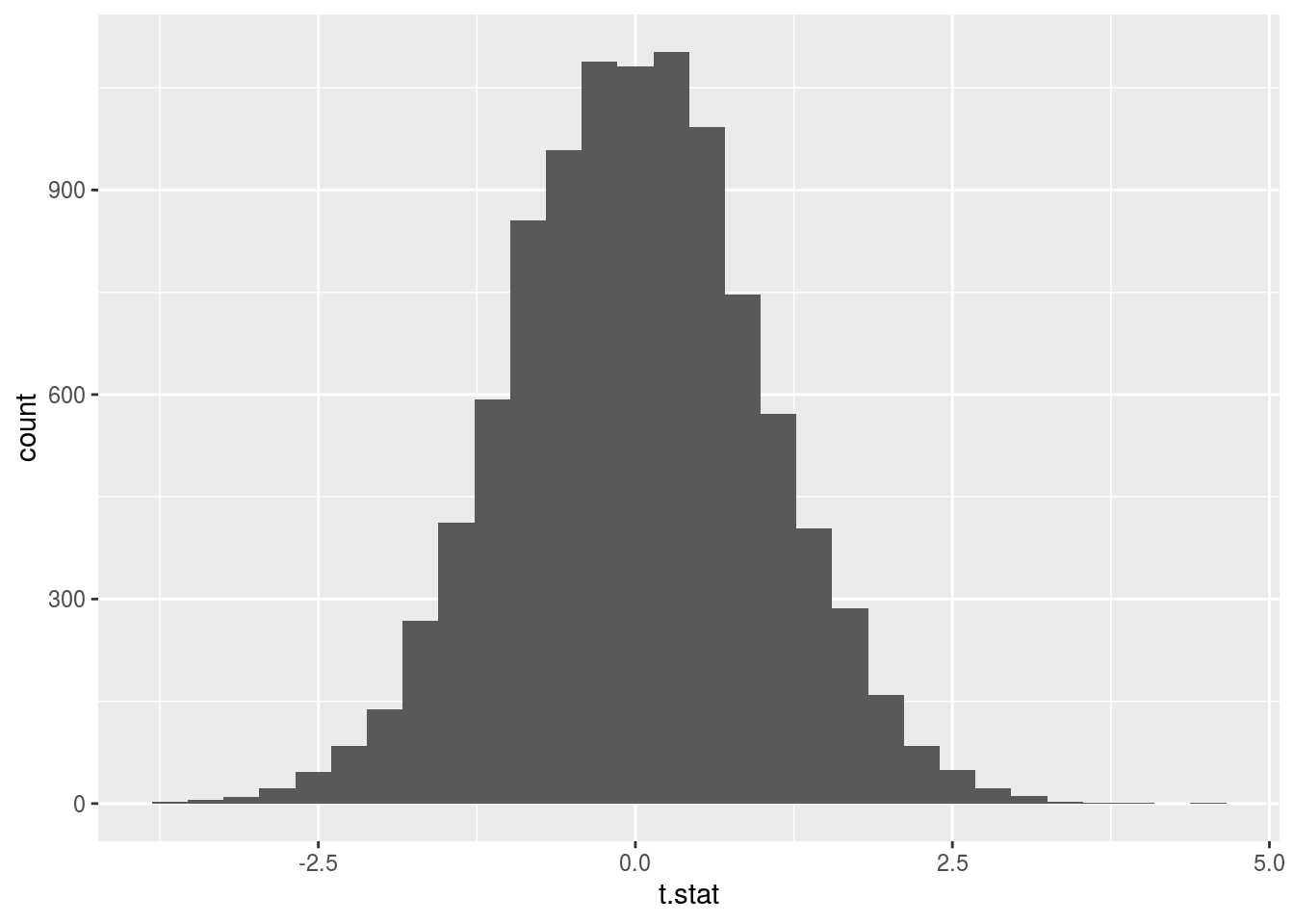nu = ((1.04^2/89 + 0.9062^2/89)^2)/(1.04^4/(89^2 * (89-1)) + 0.906^4/(89^2 * (89-1))  )
t.stat <- (9.47 - 10.1)/(sqrt(1.04^2/89 + 0.906^2/89 ))

2 * pt(-abs(t.stat), df = nu)
##  2.746046e-05
mean(abs(t.stats) > abs(t.stat))
##  1e-04

### Problem 5

A score, such as the decile score in Project 1, is said to satisfy calibration if the probability of the individual who was scored $$s$$ being actually positive is the same, regardless of their demographic.

Display a bar plot that shows the probability of actually being positive for each decile score, for Caucasians and African Americans. Does it seem that the decile scores satisfy calibration?

For each bar, display the confidence interval associated with it, using geom_errorbar. Does it now seem that the decile scores satisfy calibration?

##### Solution

We can try to see whether calibration is approximately satisfied by looking at the “decile scores” that we can obtain for the Titanic data:

titanic <- read.csv("http://guerzhoy.princeton.edu/201s20/titanic.csv")
fit <- glm(Survived ~  Age + Pclass, family = binomial, data = titanic)
titanic$decile <- round(predict(fit, newdata = titanic, type = "response")*10) titanic.surv <- titanic %>% group_by(decile, Sex) %>% summarize(Surv.Rate = mean(Survived), Surv.Total = sum(Survived), n = n()) %>% rowwise() %>% mutate(surv.min = binom.test(Surv.Total, n, p = Surv.Rate)$conf.int,
surv.max = binom.test(Surv.Total, n, p = Surv.Rate)$conf.int) %>% ungroup() %>% group_by(decile) %>% filter(n() > 1) ggplot(titanic.surv, mapping = aes(x = decile, y = Surv.Rate, fill = Sex)) + geom_bar(stat = "identity", position = "dodge") + geom_errorbar(mapping = aes(ymin = surv.min, ymax = surv.max), position = "dodge") + scale_x_continuous(breaks = c(0:10))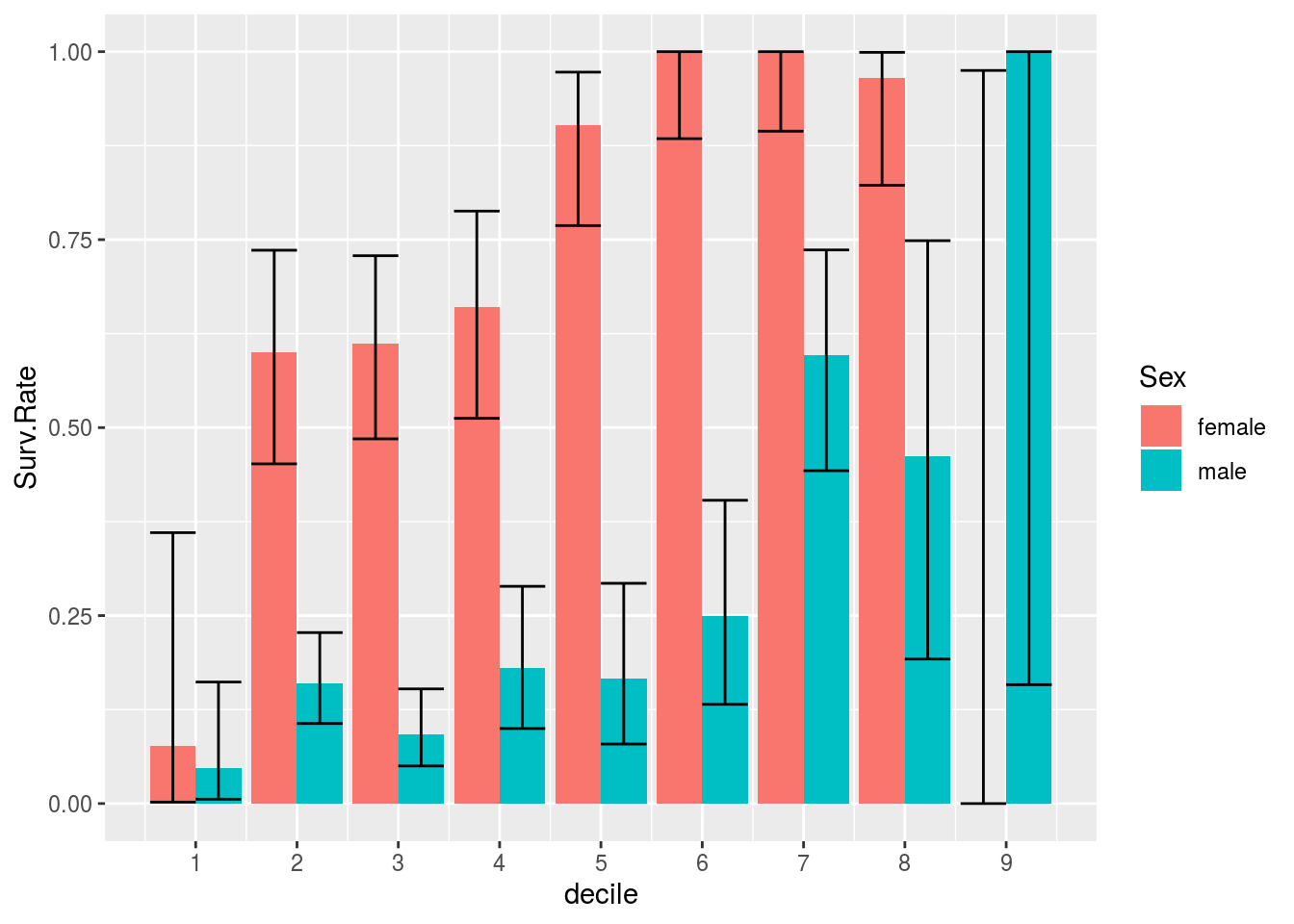We’d expect equal survival probabilities for the same deciles if there is calibration. As you can see, that is not exactly the case; furthermore, there are no male passangers at all in the top three deciles. We can try again, without including sex as a predictor: fit <- glm(Survived ~ Sex + Age + Pclass, family = binomial, data = titanic) titanic$decile <- round(predict(fit, newdata = titanic, type = "response")*10)

titanic.surv <- titanic %>% group_by(decile, Sex) %>%
summarize(Surv.Rate = mean(Survived), Surv.Total = sum(Survived), n = n()) %>%
rowwise() %>%
mutate(surv.min = binom.test(Surv.Total, n, p = Surv.Rate)$conf.int, surv.max = binom.test(Surv.Total, n, p = Surv.Rate)$conf.int) %>%
ungroup() %>%
group_by(decile) %>%
filter(n() > 1)

ggplot(titanic.surv, mapping = aes(x = decile, y = Surv.Rate, fill = Sex)) +
geom_bar(stat = "identity", position = "dodge") +
geom_errorbar(mapping = aes(ymin = surv.min, ymax = surv.max), position = "dodge") +
scale_x_continuous(breaks = c(0:10))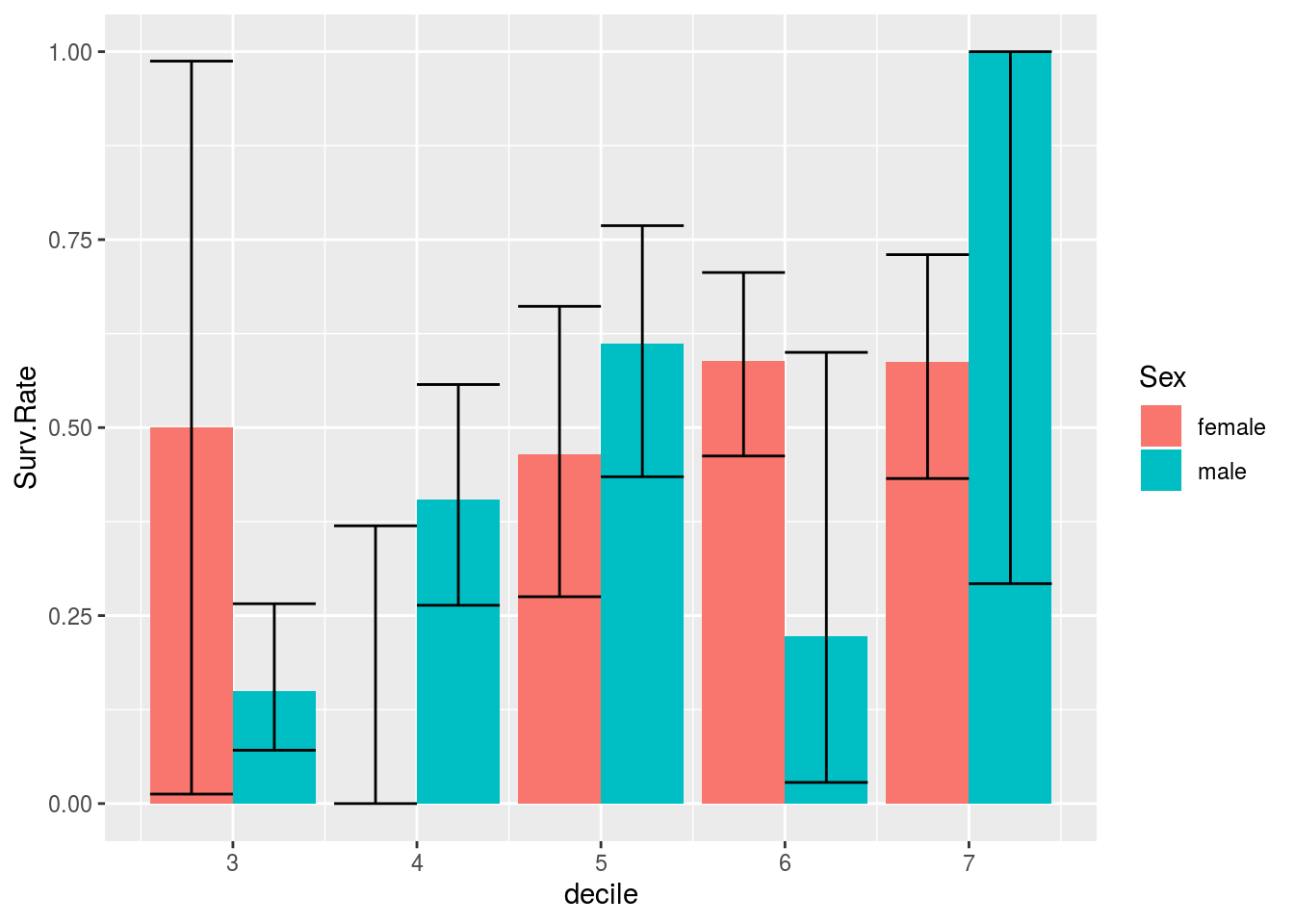We can’t reject the idea that there is calibration here as well. But since sex is a predictor of survival, we’d expect that there would be no calibration.